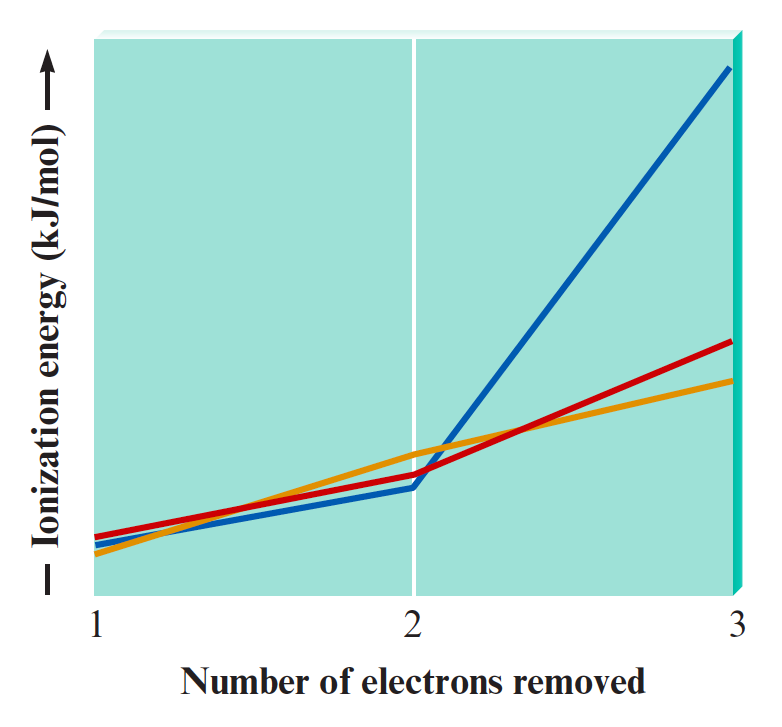# Problem: The following graph plots the first, second, and third ionization energies for Mg, Al, and Si.Without referencing the text, which plot corresponds to which element? In one of the plots, there is a huge jump in energy between I2 and I3, unlike in the other two plots. Explain this phenomenon.

###### FREE Expert Solution

Ionization energy (I. E.) is the energy required to remove an electron from a gaseous atom or ion.

First I.E. corresponds to removing thfirst valence electron from a neutral atom

Second I.E. refers to removing a second electron

Third I.E. refers to removing a third electron.

94% (369 ratings)###### Problem Details

The following graph plots the first, second, and third ionization energies for Mg, Al, and Si.Without referencing the text, which plot corresponds to which element? In one of the plots, there is a huge jump in energy between I2 and I3, unlike in the other two plots. Explain this phenomenon.

Frequently Asked Questions

What scientific concept do you need to know in order to solve this problem?

Our tutors have indicated that to solve this problem you will need to apply the Periodic Trend: Successive Ionization Energies concept. You can view video lessons to learn Periodic Trend: Successive Ionization Energies. Or if you need more Periodic Trend: Successive Ionization Energies practice, you can also practice Periodic Trend: Successive Ionization Energies practice problems.

What professor is this problem relevant for?

Based on our data, we think this problem is relevant for Professor Holme's class at ISU.

What textbook is this problem found in?

Our data indicates that this problem or a close variation was asked in Chemistry: An Atoms First Approach - Zumdahl Atoms 1st 2nd Edition. You can also practice Chemistry: An Atoms First Approach - Zumdahl Atoms 1st 2nd Edition practice problems.